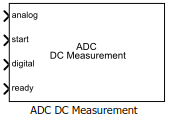Documentation

Measure DC performance metrics of ADC output

• Library:
• Mixed-Signal Blockset / ADC / Measurements & Testbenches## Description

The ADC DC Measurement block measures ADC DC performance metrics such as offset error, gain error, integral nonlinearity (INL), and differential nonlinearity (DNL). You can use ADC DC Measurement block to validate the ADC architectural models provided in Mixed-Signal Blockset™, or you can use an ADC of your own implementation.

## Ports

### Input

expand all

Data Types: `double`

External conversion start clock, specified as a scalar or a vector. The state of startindicates when the analog to digital conversion process starts.

Data Types: `double`

Converted digital signal from an ADC, specified as a scalar or a vector. The digital signal can be `BinaryVector`, `single`, `double`, `uint16``uint8`, `uint32`, or `fixdt(0,Nbits)`.

Data Types: `fixed point` | `single` | `double` | `uint8` | `uint16` | `uint32`

Indicates whether ADC conversion is complete, specified as a scalar or a vector.

Data Types: `double`

## Parameters

expand all

Number of physical bits in ADC, specified as a unitless real positive scalar. Number of bits must match the resolution specified in the ADC block. This parameter is used to calculate Recommended simulation stop time.

#### Programmatic Use

• Use `get_param(gcb,'NumBits')` to view the current Number of bits.

• Use `set_param(gcb,'NumBits',value)` to set Number of bits to a specific value.

Frequency of the start conversion clock of the ADC, specified as a real positive scalar in Hz. Start conversion frequency must match the frequency of the start conversion clock of the ADC block. This parameter is used to calculate Recommended simulation stop time.

#### Programmatic Use

• Use `get_param(gcb,'Frequency')` to view the current value of Start conversion frequency.

• Use `set_param(gcb,'Frequency',value)` to set Start conversion frequency to a specific value.

Dynamic range of ADC, specified as a 2-element vector in V. The two vector elements represent the minimum and maximum values of the dynamic range, from left to right.

#### Programmatic Use

• Use `get_param(gcb,'InputRange')` to view the current value of Input range.

• Use `set_param(gcb,'InputRange',value)` to set Input range to a specific value.

Delays measurement analysis to avoid corruption by transients, specified as a real nonnegative scalar in s.

#### Programmatic Use

• Use `get_param(gcb,'HoldOffTime')` to view the current value of Hold off time.

• Use `set_param(gcb,'HoldOffTime',value)` to set Hold off time to a specific value.

Minimum time the simulation must run for meaningful results, specified as a real positive scalar in s. Recommended simulation stop time is calculated from Number of bits and Start conversion frequency. This parameter is nontunable.

Store detailed test results to a `struct` in the base workspace for further processing. By default, this option is not selected.

Name of the variable that stores detailed test results, specified as a character string.

#### Dependencies

This parameter is only available when Output result to base workspace is selected

#### Programmatic Use

• Use `get_param(gcb,'VariableName')` to view the current value of Workspace variable name.

• Use `set_param(gcb,'VariableName',value)` to set Workspace variable name to a specific value.

Click to plot measurement result for further analysis.

expand all